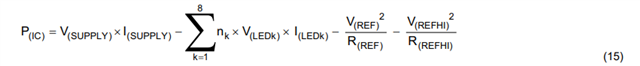If you have a related question, please click the "Ask a related question" button in the top right corner. The newly created question will be automatically linked to this question.

# TPS92638-Q1: Number of LEDs per Channel

Part Number: TPS92638-Q1

According to the data sheet, the number of LEDs per channel of the TPS92638-Q1 device is 3. Why is the channel limited to 3 LEDs? With LEDs in series being and a Vf or 3 volts, you could get up to 10-12 LEDs in series assuming a 40V Supply input. I have seen applications using this device where the number of LEDs in series was more than 3.

• Hi Greg,

The 3 LEDs is determined by the input voltage which is assumed to be Vbat 12V. Of course if the Vin is higher, the output LED could be more. But you need to calculate  thermal according to the equation:BR,

Gracie